# JP2004087857A - Equivalent circuit of voltage-controlled variable-capacitance element - Google Patents

## Info

Publication number
JP2004087857A
JP2004087857A JP2002247870A JP2002247870A JP2004087857A JP 2004087857 A JP2004087857 A JP 2004087857A JP 2002247870 A JP2002247870 A JP 2002247870A JP 2002247870 A JP2002247870 A JP 2002247870A JP 2004087857 A JP2004087857 A JP 2004087857A
Authority
JP
Japan
Prior art keywords
voltage
gate
equivalent circuit
substrate
variable capacitance
Prior art date
Legal status (The legal status is an assumption and is not a legal conclusion. Google has not performed a legal analysis and makes no representation as to the accuracy of the status listed.)
Pending
Application number
JP2002247870A
Other languages
Japanese (ja)
Inventor
Hiroki Fujimoto
Susumu Kurosawa

Original Assignee
Nec Electronics Corp
Ｎｅｃエレクトロニクス株式会社
Priority date (The priority date is an assumption and is not a legal conclusion. Google has not performed a legal analysis and makes no representation as to the accuracy of the date listed.)
Filing date
Publication date
Application filed by Nec Electronics Corp, Ｎｅｃエレクトロニクス株式会社 filed Critical Nec Electronics Corp
Priority to JP2002247870A priority Critical patent/JP2004087857A/en
Publication of JP2004087857A publication Critical patent/JP2004087857A/en
Application status is Pending legal-status Critical

• 239000000758 substrates Substances 0 abstract 3
• 239000003990 capacitor Substances 0 abstract 1
• 238000004088 simulation Methods 0 abstract 1
• 239000000126 substances Substances 0 abstract 1

## Images

•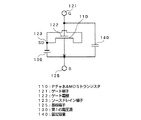••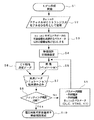•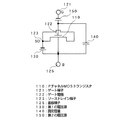•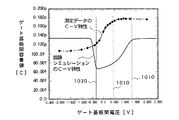•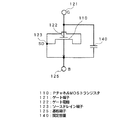•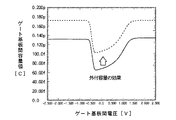•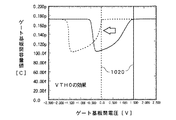•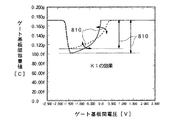•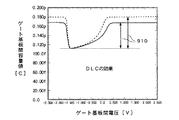•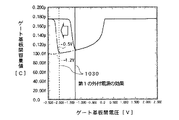•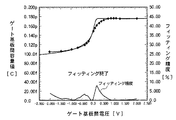•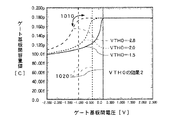•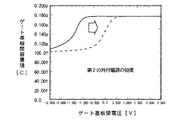••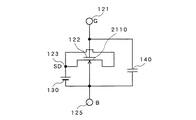•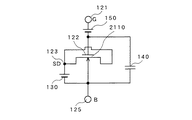•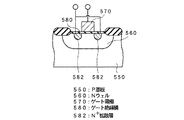•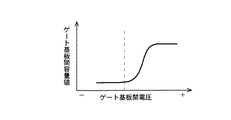•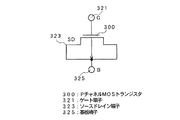•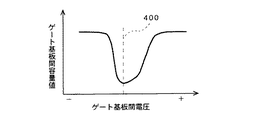## Classifications

• HELECTRICITY
• H03BASIC ELECTRONIC CIRCUITRY
• H03JTUNING RESONANT CIRCUITS; SELECTING RESONANT CIRCUITS
• H03J3/00Continuous tuning
• H03J3/20Continuous tuning of single resonant circuit by varying inductance only or capacitance only
• HELECTRICITY
• H03BASIC ELECTRONIC CIRCUITRY
• H03LAUTOMATIC CONTROL, STARTING, SYNCHRONISATION, OR STABILISATION OF GENERATORS OF ELECTRONIC OSCILLATIONS OR PULSES
• H03L7/00Automatic control of frequency or phase; Synchronisation
• H03L7/06Automatic control of frequency or phase; Synchronisation using a reference signal applied to a frequency- or phase-locked loop
• H03L7/08Details of the phase-locked loop
• H03L7/099Details of the phase-locked loop concerning mainly the controlled oscillator of the loop

## Abstract

A voltage-controlled variable capacitance element capable of simulating circuit operation characteristics including a CV characteristic of a voltage-controlled variable capacitance element with high accuracy and greatly facilitating circuit design using the voltage-controlled variable capacitance element. Is provided.
In this equivalent circuit, a gate terminal is connected to a gate electrode of a P-channel MOS transistor expressing a variable capacitance, and a fixed capacitance is provided between a substrate terminal having a substrate potential and the gate terminal. It is connected. The source and drain of the P-channel MOS transistor 110 are commonly connected to the source / drain terminal 123 and have the same potential. Between the source / drain terminal 123 and the substrate terminal 125, the substrate terminal 125 side has a positive potential. One voltage source 130 is connected.
[Selection diagram] Fig. 1

## Description


TECHNICAL FIELD OF THE INVENTION
The present invention relates to an equivalent circuit for simulating a voltage variable capacitance element that changes a capacitance value with an external voltage in order to set a resonance frequency of a voltage controlled oscillator or the like to a predetermined value.

[Prior art]
FIG. 18 is a cross-sectional view showing a variable capacitance element composed of a general accumulation mode MOS varactor. For example, an N well 560 is formed in an element formation region on the surface of a P-type substrate 550 separated by an element isolation film, and a polysilicon gate electrode 570 is formed on the surface of the element formation region via a gate oxide film 580. Is formed. Then, a pair of N-wells + A diffusion layer 582 is formed. Thereby, a MOS capacitor is formed between gate electrode 570 and N well 560 with gate insulating film 580 interposed therebetween, and gate electrode 570 and N + A variable capacitance element having the diffusion layer 582 as a contact point is configured. In the case of a normal P-channel MOS transistor, a pair of diffusion layers formed on the surface of N well + Conductive type.

FIG. 19 is a graph showing the high-frequency CV characteristics of the accumulation mode MOS varactor shown in FIG. 18, with the horizontal axis representing the voltage between the gate substrates and the vertical axis representing the capacitance between the gate substrates. The high frequency CV characteristic of the accumulation mode MOS varactor is such that when a negative voltage is applied as a gate-substrate voltage, the depletion layer on the surface of the N-well 560 spreads to the substrate 550 side, so that the gate-substrate capacitance value is reduced. The capacitance value becomes the capacitance value of the series circuit of the gate oxide film 580 and the depletion layer, and the capacitance value between the gate substrates decreases. Further, as the absolute value of the negative potential applied between the gate substrates is increased, the distance at which the width of the depletion layer spreads is saturated, so that the capacitance value between the gate substrates is also saturated to the minimum value. Conversely, as the gate-substrate voltage increases from the negative potential side to the positive potential side, the depletion layer becomes narrower and the gate-substrate capacitance value increases. As the absolute value of the positive potential applied between the gate substrates is increased, an accumulation layer is formed on the surface of N well 560, and the capacitance between the gate substrates saturates to the capacitance determined by gate oxide film 580.

As described above, the variable capacitance element includes the gate electrode 570 and the N + A capacitance element that changes the capacitance value with an external voltage in order to change the capacitance value between the gate substrates according to the voltage applied to the diffusion layer 582 and to set the resonance frequency of a voltage controlled oscillator or the like to a predetermined value Used as

Therefore, when designing the shape and size of the voltage-controlled variable capacitance element, there is a limit in evaluating all the elements and evaluating the characteristics. 2. Description of the Related Art Conventionally, an equivalent circuit of a variable capacitance element is created, and the shape and size and characteristics of the variable capacitance element are evaluated by numerical calculation using the equivalent circuit.

FIG. 20 shows a conventional equivalent circuit of this variable capacitance element, and FIG. 21 shows CV characteristics of this equivalent circuit. This conventional equivalent circuit has a structure in which a source / drain (SD) terminal 323 of a P-channel MOS transistor 300 is connected to the same potential, and the source / drain terminal 323 is connected to a substrate terminal 325. The gate electrode 321 and the substrate terminal 325 By changing the gate-substrate voltage between the above, the capacitance value between the gate substrates changes.

In a conventional equivalent circuit using a normal PMOS transistor as it is, when a voltage is applied to the gate electrode, the potential on the substrate surface changes, and accordingly, the state changes to an accumulation state, a depletion state, and an inversion state. Therefore, when the gate-to-substrate voltage is a positive potential and the storage state or the depletion layer is extended, that is, the gate-to-substrate voltage is higher than the potential (threshold voltage) shown by the broken line 400 in FIG. When it is high, a capacitance change depending on the gate-substrate potential is obtained.

[Problems to be solved by the invention]
However, when the gate-substrate voltage is lowered below the threshold voltage, minority carrier holes are supplied from the source / drain region, and the inversion layer starts to be formed. Due to the formation of the inversion layer, the capacitance value between the gate substrates increases as the voltage between the gate substrates decreases. As described above, in the case of the equivalent circuit of the conventional accumulation mode varactor, when the gate-substrate voltage falls on the negative potential side with respect to the threshold potential shown by the broken line 400 in FIG. Will happen.

Thus, as shown in FIG. 21, the CV characteristic of the conventional equivalent circuit is such that the relationship between the bias voltage for controlling the variable capacitance and the varactor capacitance reverses at the gate-substrate voltage indicated by the broken line 400 in FIG. There is a problem of doing. This reversal phenomenon becomes a problem particularly when the conventional model is used as a variable capacitor of a VCO (Voltage-Controlled Oscillator) of a PLL (Phase-Locked Loop) circuit. For example, in order to correct the phase delay (increase the frequency), the voltage (bias) between the gate and the substrate of the varactor is reduced to reduce the capacitance value. By lowering the potential, the capacitance value between the gate substrates normally decreases, and the frequency increases.

However, if the phase of the varactor is further reduced when the phase is further delayed, the varactor enters a negative potential side with respect to the potential shown by the broken line 400. Will increase. Therefore, there is a problem that the phase is delayed. Then, the PLL circuit operates to further lower the bias of the varactor, and the capacitance value between the gate substrates further increases. Such an operation is repeated, and phase control cannot be performed.

As described above, the conventional model has a problem that the bias range in which the voltage-controlled variable capacitance element (varactor) can be expressed can be used only in a narrower range on the positive potential side than the potential indicated by the broken line 400.

The present invention has been made in view of such a problem, and can simulate circuit operation characteristics including CV characteristics of a voltage-controlled variable capacitance element with high accuracy, and design a circuit using the voltage-controlled variable capacitance element. It is an object of the present invention to provide an equivalent circuit of a voltage-controlled variable capacitance element which can extremely easily perform the above.

[Means for Solving the Problems]
An equivalent circuit of the voltage controlled variable capacitance element according to the present invention includes a MOS transistor having a source and a drain connected to each other, and a first voltage source connected between a source / drain terminal of the MOS transistor and a substrate terminal. A fixed capacitance connected between the gate electrode of the MOS transistor and the substrate, and controlling the voltage by a capacitance characteristic between the gate terminal connected to the gate electrode of the MOS transistor and the substrate terminal. It is characterized in that it simulates the element characteristics of a variable capacitance element.

The equivalent circuit of the voltage-controlled variable capacitance element may further include a second voltage source connected between the gate terminal and the gate electrode.

The MOS transistor is, for example, a P-channel MOS transistor. The element characteristics of the voltage-controlled variable capacitance element to be simulated are, for example, CV characteristics between a gate substrate voltage V and a gate substrate capacitance C.

The CV characteristic can be adjusted by adjusting the capacitance value of the fixed capacitor so as to increase the capacitance value between the gate substrates as a whole. Further, by adjusting the voltage value of the first voltage source, the voltage between the gate substrates generated by the inversion layer can be adjusted to be shifted in the negative voltage direction. Further, by adjusting the voltage value of the second voltage source, it is possible to adjust the CV characteristic in the direction of increasing the gate-substrate voltage value to the positive potential side as a whole. Furthermore, the circuit characteristics of the equivalent circuit can be simulated using a Bsim3 simulator.

BEST MODE FOR CARRYING OUT THE INVENTION
Hereinafter, embodiments of the present invention will be specifically described with reference to the accompanying drawings. FIG. 1 is a circuit diagram showing an equivalent circuit of the voltage controlled variable capacitance element according to the first embodiment of the present invention. In this equivalent circuit, a gate terminal 121 is connected to a gate electrode 122 of a P-channel MOS transistor 110 expressing a variable capacitance, and a fixed capacitance 140 is connected between a substrate terminal 125 and a gate terminal 121 at a substrate potential. 110 is connected in parallel. The source and drain of the P-channel MOS transistor 110 are commonly connected to the source / drain terminal 123 and have the same potential. Between the source / drain terminal 123 and the substrate terminal 125, the substrate terminal 125 side has a positive potential. One voltage source 130 is connected.

FIG. 2 shows CV characteristics in the equivalent circuit thus configured. The horizontal axis in FIG. 2 is the voltage between the gate substrates applied between the gate terminal 121 and the substrate terminal 125, and the vertical axis is the capacitance between the gate substrates at that time. In the present invention, since the first voltage source 130 for applying a bias is connected between the source drain terminal 123 and the substrate terminal 125 so as to be in parallel with the P-channel MOS transistor 110, the inversion layer is formed. The voltage is shifted in the negative voltage direction (indicated by the white arrow in FIG. 2). That is, conventionally, as shown in FIG. 21, an inversion layer is formed at a gate-to-substrate voltage lower than the potential indicated by the broken line 400 to increase the gate-to-substrate capacitance value. The potential at which the layer is formed can be shifted to the negative potential side up to the potential indicated by the broken line 610 in FIG. Therefore, in the voltage range 600 sandwiched between the broken line 610 and the broken line 660 in FIG. 2, the CV characteristic is equivalent to the actual CV characteristic of the voltage-controlled variable capacitance element shown in FIG. The fixed capacitance 140 expresses the parasitic capacitance of the transistor, and expresses the capacitance (fringing capacitance) of the flat portion where the capacitance value between the gate substrates on the negative potential side in FIG. 19 is minimized.

In the present invention, using such an equivalent circuit as a model, the shape and dimensions of a voltage-controlled variable capacitance element that can obtain desired characteristics are obtained by a circuit simulator. A well-known model for such a circuit simulator is Bsim3, which has been developed and developed by a device group at the University of California, Berkeley. Although this Bsim model is basically a physical model, it takes bold approximations everywhere from the viewpoint of shortening calculation time and improving convergence in consideration of the characteristics as a model for a circuit simulator. Many fitting parameters are introduced to save the degradation of accuracy due to approximation.

In this case, the shift of the threshold voltage of the P-channel MOS transistor 110 caused by adding the first voltage source 130 between the source / drain terminal 123 and the substrate terminal 125 is determined by fitting a parameter expressing the threshold voltage of Bsim3. I do. Also, by changing the parameter of Bsim3 expressing the source capacitance and the drain capacitance of the normal MOS transistor model to almost zero, the influence of the capacitance other than the variable capacitance is minimized. The expression of the fringing capacitance and the like is performed by fitting with a fixed capacitance 140 connected in parallel to the P-channel MOS transistor 110.

FIG. 3 is a flowchart showing a process of creating an equivalent circuit of a MOS varactor by using Bsim3. Model creation is started (step S1), and each component of the equivalent circuit shown in FIG. 1 is assembled using a model of a P-channel MOS transistor built in Bsim3 (step S2). Then, among the Bsim3 model parameters, the capacity other than the parameter expressing the variable capacity is set to almost 0 (step S3). After that, the initial value of the equivalent circuit is set (step S4). Then, a circuit simulation is performed (step S5), and CV characteristics of the equivalent circuit shown in FIG. 1 are obtained. On the other hand, measured data of the CV characteristic of the actual device of the voltage-controlled variable capacitance element shown in FIG. 18 is obtained (step S6), and the actually measured data and the circuit simulation result are read (step S7). Is determined (step S8). If the fitting accuracy is poor (No), the values of the fixed capacitor 140 and the first voltage source 130 and the parameter of Bsim3 are adjusted (Step S9), and the circuit simulation is performed again (Step S5). The comparison between the actual measurement data and the circuit simulation result is repeated, and when it is determined that the fitting accuracy is sufficient (Yes), an equivalent circuit of the MOS varactor (variable capacitance element) is completed with the parameters at that time. . Using the equivalent circuit of the voltage-controlled variable capacitance element thus obtained, a voltage-controlled variable capacitance element having desired characteristics is designed.

FIG. 4 is a circuit diagram showing an equivalent circuit of the voltage-controlled variable capacitance element according to the second embodiment of the present invention. This embodiment is different from the first embodiment shown in FIG. 1 in that the second voltage source 150 is connected between the gate terminal 121 and the gate electrode 122 of the P-channel MOS transistor 110 on the gate electrode 122 side. That they are connected to a potential.

In the equivalent circuit of the second embodiment, the shift of the threshold voltage caused by adding the first voltage source 130 between the source / drain terminal 123 and the substrate terminal 125 is adjusted by adjusting the parameter expressing the threshold voltage of Bsim3. In addition to the above, the second voltage source 150 is connected in series between the gate terminal 121 and the gate electrode 122 to adjust the parameters of the second voltage source 150, so that the fitting can be performed more flexibly. It is possible to do. That is, in the second embodiment, the threshold voltage of the P-channel MOS transistor 110 can be controlled by the second voltage source 150 inserted in series with the gate, and the parameter for controlling the threshold value of Bsim3 can be controlled. The combination enables flexible setting. As a result, an equivalent circuit having a CV characteristic more approximate to the CV characteristic of the actual device of the variable capacitance element (varactor) in which the inversion layer does not occur can be obtained.

Next, a description will be given of a fitting effect when a simulation is performed using the circuit components used in the equivalent circuit. The effect of each circuit component of the equivalent circuit of the embodiment shown in FIG. 1 or 4 on the CV characteristic simulation is as follows. First, the external fixed capacitor 140 increases or decreases the capacitance value of the entire CV characteristic. The first voltage source 130 shifts the inversion layer generated voltage. Further, the second voltage source 150 shifts the rising position of the CV characteristic.

On the other hand, the effect of the Bsim3 model parameter on the CV characteristic simulation is as follows. First, the DLC increases or decreases the amplitude of the variable capacitance element. In addition, VTH0 shifts the rising position of the CV characteristic, and the angle of the corner of the CV characteristic can be controlled by the adjustment. Further, K1 increases or decreases the inclination angle and amplitude of the rise of the CV characteristic.

However, parts and model parameters have a dependency relationship with each other. For this reason, the above description describes the places where the simulation results apparently change the characteristics the most when the values are changed, and the characteristic relationship with each component does not correspond one-to-one.

Fitting is performed using such characteristics of each parameter. The fitting is a process in which the values of the components of the equivalent circuit are adjusted to the CV characteristics obtained by high-frequency measurement of the actual device of the variable capacitance element so that the result of the circuit simulation matches the required accuracy. That is. Hereinafter, a specific fitting method will be described.

First, the basic CV characteristics of the P-channel MOS transistor are as shown in FIG. FIG. 5 shows a simulation result (solid line) of the conventional equivalent circuit shown in FIG. 20, that is, an equivalent circuit of a basic P-channel MOS transistor (L = 0.16 μm, W = 100 μm), and a voltage-controlled variable capacitance element. And CV characteristic measurement data (dotted chain line, Δ) of the actual device. As described above, the CV characteristic of the P-channel MOS transistor is such that the capacitance between gate substrates increases due to the inversion layer generated voltage (indicated by line 1030) on the negative potential side (about -0.3 V) due to the influence of the inversion layer. Has occurred. In addition, the capacitance rising potential (indicated by line 1010) is large and on the positive potential side, and the overall capacitance is small.

Next, among the Bsim3 model parameters, the parameter expressing the capacity is set to almost zero (0). The Bsim3 model parameters other than the parameter expressing the variable capacitance include CGSO (parasitic capacitance between gate and source), CGDO (parasitic capacitance between gate and drain), CJ (fringe electric field capacitance), and CJSW (zero bias bulk junction side surface capacitance). ). These parameters are reduced as long as they are not affected by the simulation. This is to prevent an unintended operation from occurring due to the value of the Bsim3 parameter (particularly, a parameter expressing capacitance) because the structure of the MOS variable capacitance element is different from that of the P-channel MOS transistor. The difference in structure is that the source / drain diffusion layer of the variable capacitance element formed in the N well on the substrate surface is N + In the case of a P-channel MOS transistor, the source / drain formed on the surface of the N-well has the same potential as the source / drain diffusion layer. + That is, it is a diffusion layer.

Next, a description will be given of a simulation result when an external fixed capacitor 140 is added to a conventional equivalent circuit (FIG. 20: only the P-channel transistor 300) as shown in FIG. In FIG. 6, the same components as those in FIG. 1 are denoted by the same reference numerals. FIG. 7 shows CV characteristics obtained by circuit simulation of the equivalent circuit shown in FIG. In FIG. 7, the solid line is the simulation result when there is no external capacitance 140, and the broken line is the simulation result when the external capacitance 140 having a capacitance of 0.07 pF is added. However, in the Bsim3 model, parameters other than the parameter expressing the variable capacity of the Bsim3 model parameters are almost zero.

As shown in FIG. 7, when an external capacitor 140 is added, the capacitance between gate substrates increases as a whole (at all gate-substrate voltages).

Next, a simulation result by adjusting the model parameter of Bsim3 will be described. VTH0 of Bsim3 is a parameter for adjusting the threshold voltage. As shown in FIG. 8, when the value of VTH0 is a negative value, the gate-substrate voltage at the position where the capacitance rises (indicated by line segment 1020) shifts in the negative voltage direction. In the figure, the broken line shows the case where VTH0 is adjusted to about -1.5.

K1 is a coefficient for the threshold value of the model. As shown in FIG. 9, when the value of K1 is increased, the slope 800 of the CV characteristic becomes gentle (decreases gradually) as indicated by a broken line in the figure, and the capacitance value decreases from the maximum value. The indicated amplitude 810 decreases. In the drawing, the broken line is obtained by adjusting K1 to about 1.1.

DLC is a gate length offset fitting parameter from CV characteristics. As shown in FIG. 10, when the value of DLC is decreased, as shown by the broken line in the figure, the amplitude 910 indicating the increase in the maximum capacitance value in the waveform of the CV characteristic increases. In the figure, the dashed line indicates that DLC is about 2.0 × 10 -8 It is adjusted to.

Next, the effect of the addition of the first voltage source 130 on the simulation will be described. As shown in FIG. 1, when an external first voltage source 130 is added to the equivalent circuit of FIG. 6, the CV characteristics obtained by the circuit simulation are as shown in FIG. In FIG. 11, the solid line is the CV characteristic by the circuit simulation when the first voltage source is not provided (the same as the broken line in FIG. 7), and the dashed line and the broken line are the case where the first voltage source 130 is added. The dashed-dotted line is the simulation result when -0.5 V is applied as the first voltage source 130, and the broken line is the simulation result when -1.2V is applied. As shown in FIG. 11, by adding the first voltage source 130, the inversion layer generated voltage (indicated by the line segment 1030) is out of the range of the varactor control voltage (in this case, -1.5 V or less). Up to the negative voltage.

By repeating the steps of (1) making the parameter expressing the capacity almost zero, (2) adding the external capacity 140, and (3) adjusting the Bsim3 model parameters, the measurement data is obtained by adjusting the parameters. The parameters are adjusted until the difference between the simulation result and the simulation result satisfies the required accuracy (for example, within ± 10%).

FIG. 12 shows the CV characteristic at the end of the fitting, with the horizontal axis representing the voltage between the gate substrates and the vertical axis representing the capacitance between the gate substrates. In the figure, ◆ indicates the CV characteristic of the measurement data of the actual device, and the solid line indicates the CV characteristic of the equivalent circuit (shown in FIG. 1) whose parameters were obtained by circuit simulation. In the figure, the fitting accuracy is also shown. As shown in FIG. 12, the measured data and the simulation result match with high accuracy, and it can be seen that the equivalent circuit of the first embodiment of the present invention has high fitting accuracy (small error).

FIG. 13 is a graph showing the effect of VTH0 on CV characteristics. When VTH0 of the model parameter of Bsim3 is set to a negative value and its absolute value is increased, the rising position 1020 of the gate-substrate capacitance value shifts to the negative potential side and the angle of the corner 1010 of the CV characteristic waveform. Changes so as to be gentle. As shown in FIG. 12, the simulation result of the equivalent circuit shown in FIG. 1 shows that the corner of the waveform of the CV characteristic has a smaller curvature and is steeper than the measured data, and the fitting accuracy is slightly poor in this part. On the other hand, as shown in FIG. 13, by setting VTH0 to a negative value, the curvature of the corner portion 1010 can be increased to make it smooth. However, as described above, by setting VTH0 to a negative value, the rising position 1020 of the capacitance is shifted to the negative potential side.

Therefore, a second voltage source 150 is provided as in the equivalent circuit of the second embodiment shown in FIG. FIG. 14 shows a simulation result (dashed line) of the CV characteristic when approximately +1.6 V is applied as the second voltage source 150, in comparison with a case where the second voltage source 150 is not provided (solid line). As shown in FIG. 14, the provision of the second voltage source 150 has the effect of shifting the rising position to the positive potential side, and cancels the voltage at the rising position 1020 of the capacitor shifted to the negative potential side by VTH0. There is. Therefore, by providing the second voltage source 150, the entire pattern of the CV characteristic can be shifted to the positive potential side of the gate-substrate voltage while maintaining the pattern shape of the CV characteristic.

The parameters K1 and DLC other than the model parameter VTH0 of Bsim3 also operate the amplitude and capacitance change of the CV characteristic, but also change the rising position 1020 of the capacitance similarly to VTH0. Also in this case, by providing the second voltage source 150, a change in the rise position 1020 of the capacitance when these parameters are operated can be canceled, the fitting operation can be performed flexibly, and the fitting operation is facilitated. become.

FIG. 15 is a graph showing the fitting accuracy when fitting is performed as described above in the equivalent circuit of the second embodiment shown in FIG. As shown in FIG. 15, the CV characteristic (◆) of the actual device of the variable capacitance element and the CV characteristic based on the simulation result of the equivalent circuit match very well, and the fitting accuracy is extremely high (the error is extremely small).

As described above, the equivalent circuit of the present invention has a structure in which the first voltage source 130 is connected between the source / drain terminal 123 and the substrate terminal 125 so as to be in parallel with the P-channel MOS transistor 110. As shown in (1), the bias voltage generated by the inversion layer can be shifted in the negative voltage direction from the control voltage range 600 of the voltage-controlled variable capacitance element (varactor) to the outside 610, and the normal MOS transistor model in Bsim3 In order to prevent the influence of the source capacitance and the drain capacitance of Bsim3, the parameters expressing the source capacitance and the drain capacitance of Bsim3 are almost zero, and the expression of the fringing capacitance and the like is performed by connecting the fixed capacitance 140 in parallel between the gate substrates. Fitting, and by providing a first voltage source 130, That the threshold voltage of the channel MOS transistor changes, can be adjusted by the parameters that control the threshold voltage of Bsim3.

Also, by inserting the second voltage source 150 in series with the gate, it becomes possible to control the threshold voltage of the P-channel MOS transistor, and it is possible to perform flexible fitting by combining with the parameter for controlling the threshold value of Bsim3. Becomes possible.

In the equivalent circuit according to the present invention, the conductivity type of the MOS transistor 110 is not limited to the P channel, but may be an N channel, and an appropriate MOS transistor can be adopted according to the variable capacitance element to be simulated. FIG. 16 is a circuit diagram showing an embodiment in which an N-channel MOS transistor 2110 is used instead of the P-channel MOS transistor 110 in the embodiment shown in FIG. In this equivalent circuit, the polarity of the first voltage source 130 is inverted. This embodiment also has the same functions and effects as the embodiment shown in FIG.

FIG. 17 is a circuit diagram showing an embodiment in which an N-channel MOS transistor 2110 is used instead of the P-channel MOS transistor 110 in the embodiment shown in FIG. In this equivalent circuit, the polarities of the first voltage source 130 and the second voltage source 150 are inverted. This embodiment also has the same functions and effects as the embodiment shown in FIG.

【The invention's effect】
As described in detail above, in the conventional equivalent circuit, as shown in the CV characteristic shown in FIG. 21, when the voltage between the gate and the substrate enters the negative potential side of the threshold value, an inversion layer is formed to increase the capacitance between the gate and the substrate. On the other hand, according to the equivalent circuit of the present invention, as shown in FIG. 2, the CV characteristic causes the bias voltage generated by the inversion layer to fall outside the control voltage range of the voltage-controlled variable capacitance element. The voltage can be shifted to the negative potential side up to the (lower potential side) voltage. As a result, a CV characteristic equivalent to the actual CV characteristic of the voltage-controlled variable capacitance element can be obtained. Therefore, by using the equivalent circuit of the present invention, it is possible to simulate the circuit operation characteristics including the CV characteristics of the voltage-controlled variable capacitance element as an actual device with extremely high accuracy. It greatly contributes to speeding up circuit design and cost reduction using a circuit.
[Brief description of the drawings]
FIG. 1 is a circuit diagram showing an equivalent circuit of a voltage-controlled variable capacitance element according to a first embodiment of the present invention.
FIG. 2 is a graph showing CV characteristics of the equivalent circuit according to the embodiment.
FIG. 3 is a flowchart illustrating the operation of the present embodiment.
FIG. 4 is a circuit diagram showing an equivalent circuit of a voltage-controlled variable capacitance element according to a second embodiment of the present invention.
5 is a graph showing a comparison between CV characteristics of a simulation result of the conventional equivalent circuit shown in FIGS. 20 and 21 and measurement data of CV characteristics of an actual device.
FIG. 6 is a circuit diagram showing an equivalent circuit to which an external fixed capacitor 140 is added.
FIG. 7 is a graph showing the effect of an external fixed capacitor 140 on CV characteristics.
FIG. 8 is a graph showing the effect of a Bsim3 parameter VTH0 on CV characteristics.
FIG. 9 is a graph showing the effect of a Bsim3 parameter K1 on CV characteristics.
FIG. 10 is a graph showing the effect of a Bsim3 parameter DLC on CV characteristics.
FIG. 11 is a graph showing the influence of the first voltage source on the CV characteristic.
FIG. 12 is a graph showing the fitting accuracy of the equivalent circuit of the first embodiment.
FIG. 13 is a graph showing the effect of a Bsim3 parameter VTH0 on CV characteristics.
FIG. 14 is a graph showing the effect of a second voltage source 150 on CV characteristics.
FIG. 15 is a graph showing the fitting accuracy of the equivalent circuit of the second embodiment.
FIG. 16 is a circuit diagram showing an equivalent circuit of a voltage-controlled variable capacitance element according to a third embodiment of the present invention.
FIG. 17 is a circuit diagram showing an equivalent circuit of a voltage-controlled variable capacitance element according to a fourth embodiment of the present invention.
FIG. 18 is a cross-sectional view illustrating a structure of a voltage-controlled variable capacitance element.
FIG. 19 is a graph showing CV characteristics of the voltage controlled variable capacitance element.
FIG. 20 is a circuit diagram showing a conventional equivalent circuit.
FIG. 21 is a graph showing CV characteristics of the conventional equivalent circuit.
[Explanation of symbols]
110: P-channel MOS transistor
121: gate terminal
122: Gate electrode
123: source drain terminal
125: Board terminal
130: first voltage source
140: fixed capacity
150: second voltage source
300: P-channel MOS transistor
321: Gate terminal
323: source drain terminal
325: Board terminal
550: P substrate
560: N well
570: Gate electrode
580: Gate insulating film
582: N + Diffusion layer
2110: N-channel MOS transistor

## Claims (7)

1. A MOS transistor having a source and a drain connected to each other, a first voltage source connected between a source / drain terminal of the MOS transistor and a substrate terminal, and a gate between the gate electrode of the MOS transistor and the substrate. Having a fixed capacitance connected thereto, and simulating element characteristics of the voltage controlled variable capacitance element by capacitance characteristics between the gate terminal to which the gate electrode of the MOS transistor is connected and the substrate terminal. An equivalent circuit of a voltage controlled variable capacitance element characterized by the above-mentioned.
2. 2. The equivalent circuit of claim 1, further comprising a second voltage source connected between the gate terminal and the gate electrode. 3.
3. 3. The equivalent circuit according to claim 1, wherein the MOS transistor is a P-channel MOS transistor.
4. 4. The device characteristic of the voltage controlled variable capacitance element to be simulated is a CV characteristic between a gate substrate voltage V and a gate substrate capacitance C. 4. The device according to claim 1, wherein: The equivalent circuit of the voltage controlled variable capacitance element of FIG.
5. The voltage control variable capacitance element according to claim 4, wherein the CV characteristic is adjusted so as to increase the gate-substrate capacitance value as a whole by adjusting the capacitance value of the fixed capacitance. Equivalent circuit.
6. 6. The method according to claim 4, wherein the voltage value of the first voltage source is adjusted so that the voltage between the gate substrates generated by the inversion layer is shifted in the negative voltage direction. An equivalent circuit of the described voltage-controlled variable capacitance element.
7. 7. The method according to claim 4, wherein the voltage value of the second voltage source is adjusted so that the CV characteristic is adjusted so as to increase the gate-substrate voltage value to the positive potential side as a whole. An equivalent circuit of the voltage controlled variable capacitance element according to any one of the above.
JP2002247870A 2002-08-27 2002-08-27 Equivalent circuit of voltage-controlled variable-capacitance element Pending JP2004087857A (en)

## Priority Applications (1)

Application Number Priority Date Filing Date Title
JP2002247870A JP2004087857A (en) 2002-08-27 2002-08-27 Equivalent circuit of voltage-controlled variable-capacitance element

## Applications Claiming Priority (2)

Application Number Priority Date Filing Date Title
JP2002247870A JP2004087857A (en) 2002-08-27 2002-08-27 Equivalent circuit of voltage-controlled variable-capacitance element
US10/647,463 US20040046600A1 (en) 2002-08-27 2003-08-26 Equivalent circuit of voltage-controlled variable capacitive element

## Publications (1)

Publication Number Publication Date
JP2004087857A true JP2004087857A (en) 2004-03-18

# Family

## Family Applications (1)

Application Number Title Priority Date Filing Date
JP2002247870A Pending JP2004087857A (en) 2002-08-27 2002-08-27 Equivalent circuit of voltage-controlled variable-capacitance element

## Country Status (2)

US (1) US20040046600A1 (en)
JP (1) JP2004087857A (en)

## Cited By (1)

* Cited by examiner, † Cited by third party
Publication number Priority date Publication date Assignee Title
JP2006042197A (en) * 2004-07-29 2006-02-09 Ricoh Co Ltd Filter circuit and d/a converter

## Families Citing this family (13)

* Cited by examiner, † Cited by third party
Publication number Priority date Publication date Assignee Title
US7068089B2 (en) * 2004-05-28 2006-06-27 Wionics Research Digitally programmable I/Q phase offset compensation
US7761823B2 (en) * 2007-05-15 2010-07-20 Advanced Micro Devices, Inc. Method for adjusting a transistor model for increased circuit simulation accuracy
EP2354882B1 (en) * 2010-02-10 2017-04-26 Nxp B.V. Switchable current source circuit and method
CN101938254A (en) * 2010-08-24 2011-01-05 上海集成电路研发中心有限公司 Mixer
US8498094B2 (en) * 2011-05-05 2013-07-30 Eta Semiconductor Inc. Semiconductor variable capacitor
US9401436B2 (en) 2011-05-05 2016-07-26 Qualcomm Incorporated Multiple control transcap variable capacitor
US10156455B2 (en) 2012-06-05 2018-12-18 Apple Inc. Context-aware voice guidance
US9418672B2 (en) 2012-06-05 2016-08-16 Apple Inc. Navigation application with adaptive instruction text
US10176633B2 (en) 2012-06-05 2019-01-08 Apple Inc. Integrated mapping and navigation application
US9052197B2 (en) 2012-06-05 2015-06-09 Apple Inc. Providing navigation instructions while device is in locked mode
US9997069B2 (en) 2012-06-05 2018-06-12 Apple Inc. Context-aware voice guidance
US8983778B2 (en) 2012-06-05 2015-03-17 Apple Inc. Generation of intersection information by a mapping service

## Cited By (2)

* Cited by examiner, † Cited by third party
Publication number Priority date Publication date Assignee Title
JP2006042197A (en) * 2004-07-29 2006-02-09 Ricoh Co Ltd Filter circuit and d/a converter
JP4596847B2 (en) * 2004-07-29 2010-12-15 株式会社リコー Filter circuit and D / A converter

## Also Published As

Publication number Publication date
US20040046600A1 (en) 2004-03-11

## Similar Documents

Publication Publication Date Title
US6294962B1 (en) Circuit(s), architecture and method(s) for operating and/or tuning a ring oscillator
US9046552B2 (en) On-die trim-able passive components for high volume manufacturing
US6448840B2 (en) Adaptive body biasing circuit and method
US20030055621A1 (en) Hot carrier circuit reliability simulation
US3806741A (en) Self-biasing technique for mos substrate voltage
CN1985440B (en) Apparatus and method for adjusting performance of integrated circuits
US6798011B2 (en) Multi-terminal MOS varactor
JP2990863B2 (en) Oscillation circuit
CN1301588C (en) Temperature compensation oscillator
US7263477B2 (en) Method and apparatus for modeling devices having different geometries
EP1327944A1 (en) Method for modeling and making an integrated circuit having at least an insulated gate field effect transistor and corresponding integrated circuit
US8461891B1 (en) Voltage compensated integrated circuits
US7222060B2 (en) Circuit simulation apparatus incorporating diffusion length dependence of transistors and method for creating transistor model
JP4257823B2 (en) Semiconductor device and capacitance measuring method
Ramirez-Angulo et al. Modeling multiple-input floating-gate transistors for analog signal processing
US4795964A (en) Method and apparatus for measuring the capacitance of complementary field-effect transistor devices
US7567891B1 (en) Hot-carrier device degradation modeling and extraction methodologies
US8089324B2 (en) Varactor-free amplitude controlled oscillator(ACO) for system on chip and system on card Xtaless clock SOC
US6133799A (en) Voltage controlled oscillator utilizing threshold voltage control of silicon on insulator MOSFETS
Maloberti Analog design for CMOS VLSI systems
CN102361449A (en) Apparatus and methods for adjusting performance of integrated circuits
JPH07154221A (en) Delay circuit
US7586347B1 (en) Clock generator with self-bias bandwidth control
US7358823B2 (en) Programmable capacitors and methods of using the same
JP2013516836A (en) Temperature stable oscillation circuit with frequency-current feedback